# microsoft 98-381 practice test

## Introduction to Programming Using Python

##### Question 1

HOTSPOT
You find errors while evaluating the following code. Line numbers are included for reference only.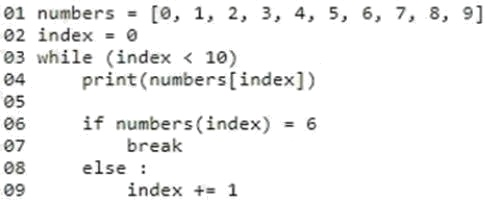You need to correct the code at line 03 and line 06.
How should you correct the code? Use the drop-down menus to select the answer choice that answers each question based
on the information presented in the code segment.
NOTE: Each correct selection is worth one point.
Hot Area: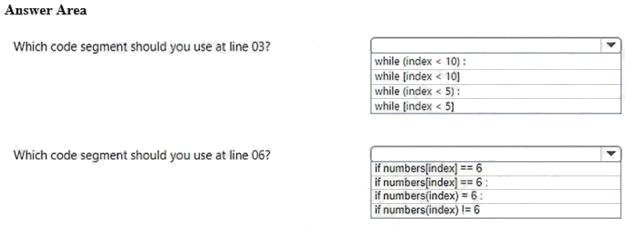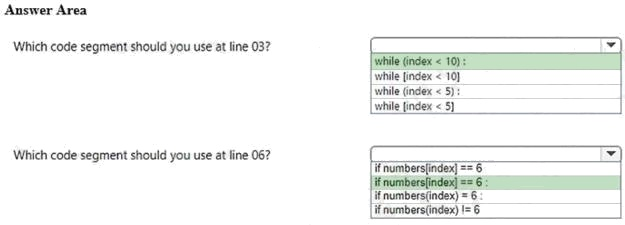Explanation:
References:
https://www.w3resource.com/python/python-while-loop.php

Discussions
0 / 1000

##### Question 2

HOTSPOT
The ABC company is building a basketball court for its employees to improve company morale.
You are creating a Python program that employees can use to keep track of their average score.
The program must allow users to enter their name and current scores. The program will output the user name and the users
average score. The output must meet the following requirements: The user name must be left-aligned.If the user name has fewer than 20 characters, additional space must be added to the right.The average score must have three places to the left of the decimal point and one place to the right of the decimal(XXX.X).
How should you complete the code? To answer, select the appropriate code segments in the answer area.
NOTE: Each correct selection is worth one point.
Hot Area: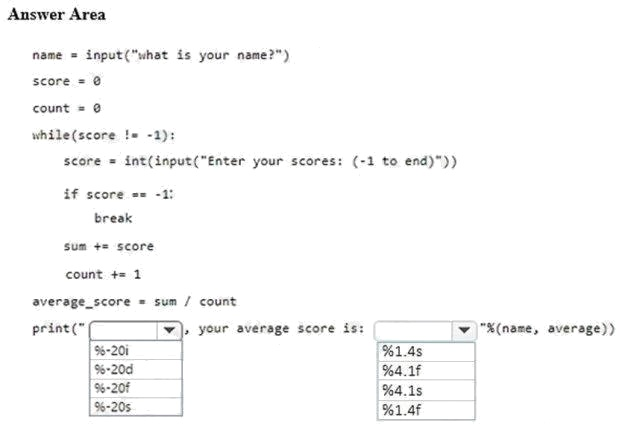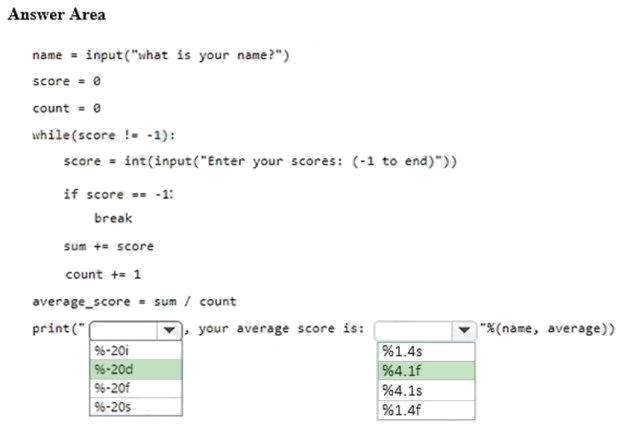Explanation:
References:
https://www.python-course.eu/python3_formatted_output.php

Discussions
0 / 1000

##### Question 3

You are creating a Python program that shows a congratulation message to employees on their service anniversary.
You need to calculate the number of years of service and print a congratulatory message.
You have written the following code. Line numbers are included for reference only.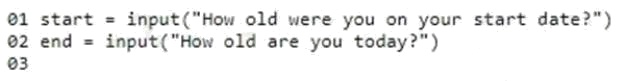You need to complete the program.
Which code should you use at line 03?

• A. print("Congratulations on" + (int(end)-int(start)) + "years of service!")
• B. print("Congratulations on" + str(int(end)-int(start)) + "years of service!")
• C. print("Congratulations on" + int(end - start) + "years of service!")
• D. print("Congratulations on" + str(end - start)) + "years of service!")

B

Explanation:
int must be converted to string

Discussions
0 / 1000

##### Question 4

You develop a Python application for your school.
You need to read and write data to a text file. If the file does not exist, it must be created. If the file has content, the content
must be removed.
Which code should you use?

• A. open(“local_data”, “r”)
• B. open(“local_data”, “r+”)
• C. open(“local_data”, “w+”)
• D. open(“local_data”, “w”)

C

Explanation:
Modes 'r+', 'w+' and 'a+' open the file for updating (reading and writing). Mode 'w+' truncates the file.
References:
https://docs.python.org/2/library/functions.html https://pythontips.com/2014/01/15/the-open-function-explained/

Discussions
0 / 1000

##### Question 5

DRAG DROP
You are writing a Python program to perform arithmetic operations.
You create the following code: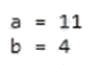What is the result of each arithmetic expression? To answer, drag the appropriate expression from the column on the left to
its result on the right. Each expression may be used once, more than once, or not at all.
Select and Place: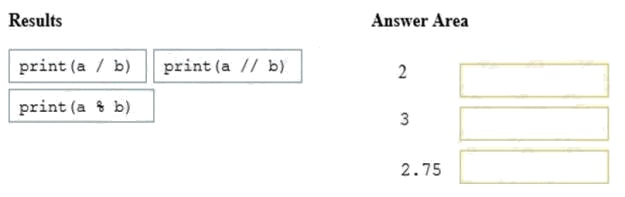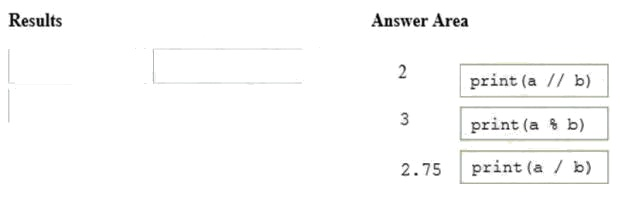Explanation:
References:
https://www.w3resource.com/python/python-operators.php

Discussions
0 / 1000

##### Question 6

HOTSPOT
You create a function to calculate the power of a number by using Python.
You need to ensure that the function is documented with comments.
You create the following code. Line numbers are included for reference only.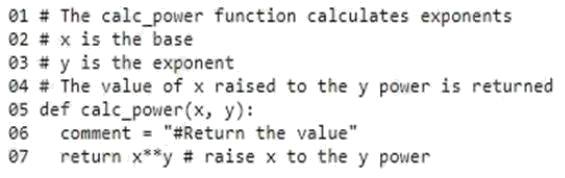For each of the following statements, select Yes if the statement is true. Otherwise, select No.
Hot Area: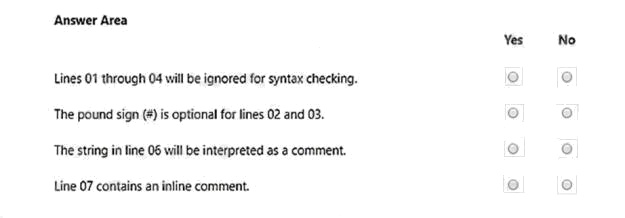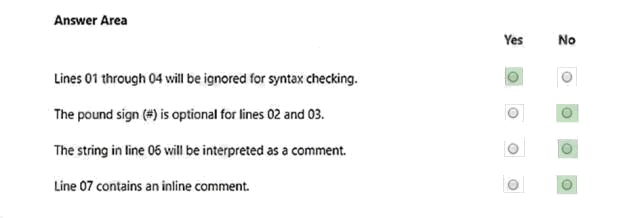Explanation:
References:
https://www.w3resource.com/python/python-string.php

Discussions
0 / 1000

##### Question 7

You develop a Python application for your company.
You need to accept input from the user and print that information to the user screen.
You have started with the following code. Line numbers are included for reference only.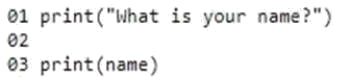Which code should you write at line 02?

• A. name = input
• B. input(“name”)
• C. input(name)
• D. name = input()

B

Discussions
0 / 1000

##### Question 8

HOTSPOT
You are developing a Python application for your company.
You write the following code: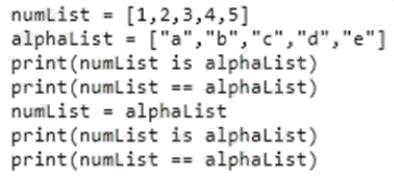Use the drop-down menus to select the answer choice that answers each question based on the information presented in
the code segment.
Hot Area: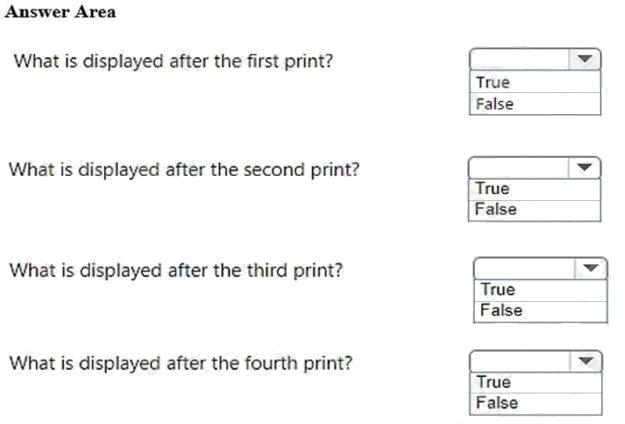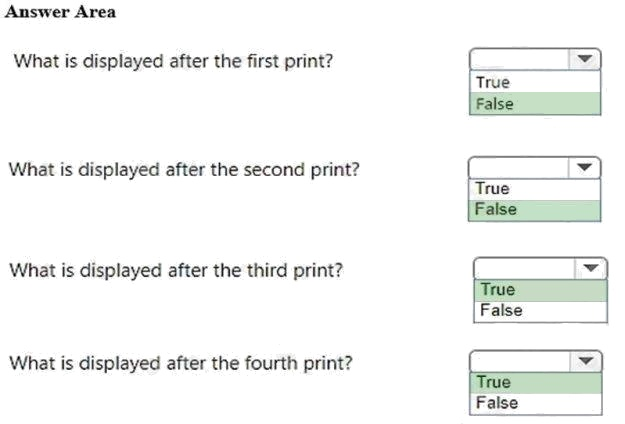Explanation:
References:
https://www.w3resource.com/python/python-list.php

Discussions
0 / 1000

##### Question 9

HOTSPOT
You create the following program to locate a conference room and display the room name. Line numbers are included for
reference only.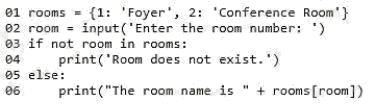Colleagues report that the program sometimes produces incorrect results.
You need to troubleshoot the program. Use the drop-down menus to select the answer choice that answers each question
based on the information presented in the code segment.
Hot Area: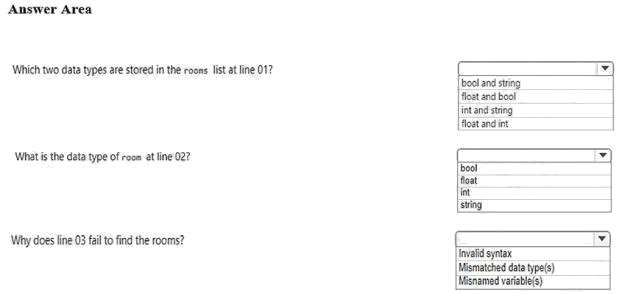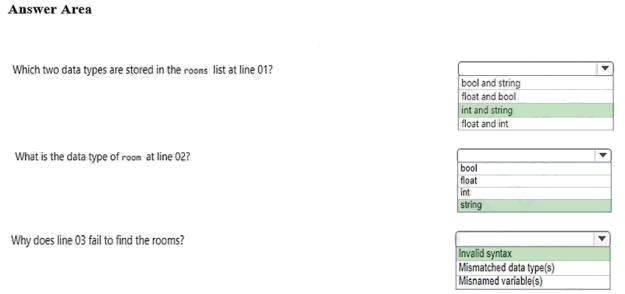Explanation:
References:
https://www.w3resource.com/python/python-data-type.php https://www.w3resource.com/python/python-if-else-
statements.php

Discussions
0 / 1000

##### Question 10

You are creating a function that manipulates a number. The function has the following requirements:
A float is passed into the functionThe function must take the absolute value of the floatAny decimal points after the integer must be removedWhich two math functions should you use? Each correct answer is part of the solution. (Choose two.)

• A. math.fmod(x)
• B. math.frexp(x)
• C. math.floor(x)
• D. math.ceil(x)
• E. math.fabs(x)

C E

Explanation:
C: math.floor(x) returns the largest integer less than or equal to x. E: math.fabs(x) returns the absolute value of x.
A: math.fmod() takes two variables
B: math.frexp(x) returns the mantissa and exponent of x as the pair (m, e). m is a float and e is an integer D: math.ceil(x)
returns the smallest integer greater than or equal to x
References:
https://docs.python.org/2/library/math.html#number-theoretic-and-representation-functions
https://docs.python.org/3/library/math.html

Discussions
0 / 1000

##### Question 11

You are creating a function that reads a data file and prints each line of the file.
You write the following code. Line numbers are included for reference only.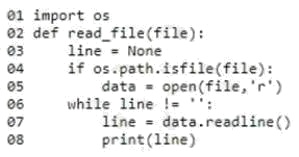The code attempts to read the file even if the file does not exist.
You need to correct the code.
Which three lines have indentation problems? Each correct answer presents part of the solution. (Choose three.)

• A. Line 01
• B. Line 02
• C. Line 03
• D. Line 04
• E. Line 05
• F. Line 06
• G. Line 07
• H. Line 08

F G H

Discussions
0 / 1000

##### Question 12

HOTSPOT
The ABC organics company needs a simple program that their call center will use to enter survey data for a new coffee
variety.
The program must accept input and return the average rating based on a five-star scale. The output must be rounded to two
decimal places.
You need to complete the code to meet the requirements.
How should you complete the code? To answer, select the appropriate code segments in the answer area.
NOTE: Each correct selection is worth one point.
Hot Area: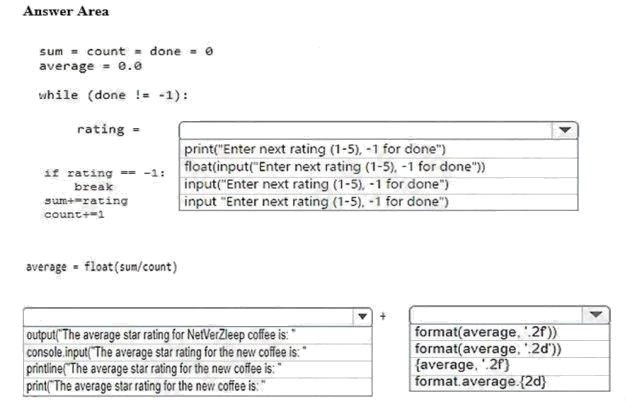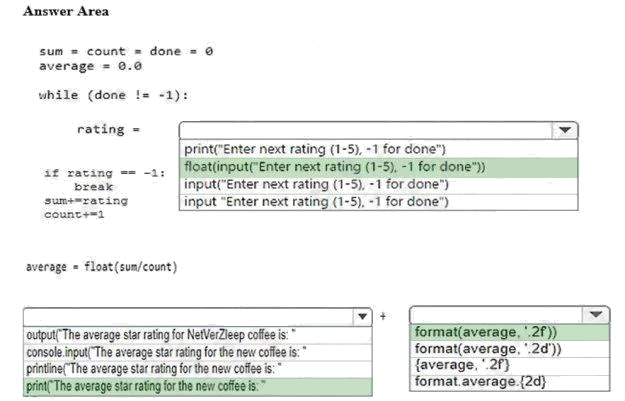Explanation:
References:
https://www.w3resource.com/python/python-format.php#num

Discussions
0 / 1000

##### Question 13

HOTSPOT
The ABC company needs a way to find the count of particular letters in their publications to ensure that there is a good
balance. It seems that there have been complaints about overuse of the letter e. You need to create a function to meet the
requirements.
How should you complete this code? To answer, select the appropriate code segments in the answer area.
NOTE: Each correct selection is worth one point.
Hot Area: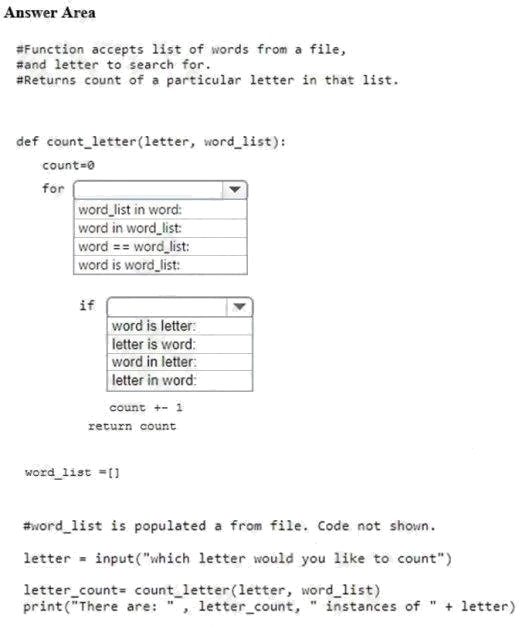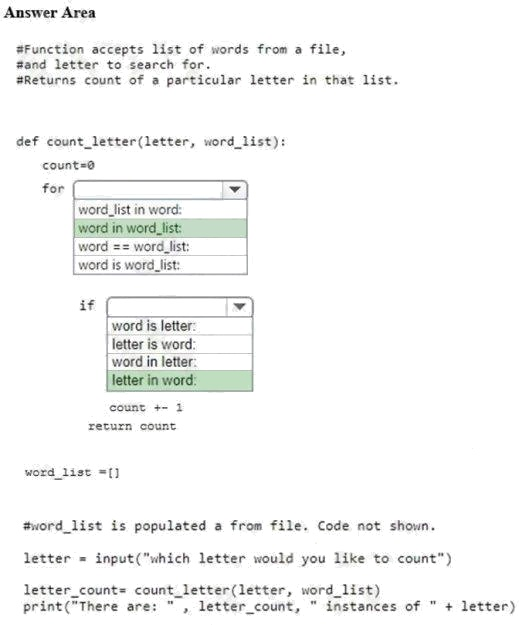Explanation:
References:
https://www.w3resource.com/python/python-for-loop.php

Discussions
0 / 1000

##### Question 14

HOTSPOT
You are designing a decision structure to convert a students numeric grade to a letter grade. The program must assign a
letter grade as specified in the following table: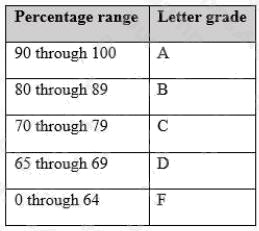For example, if the user enters a 90, the output should be, Your letter grade is A. Likewise, if a user enters an 89, the
How should you complete the code? To answer, select the appropriate code segments in the answer area.
Hot Area: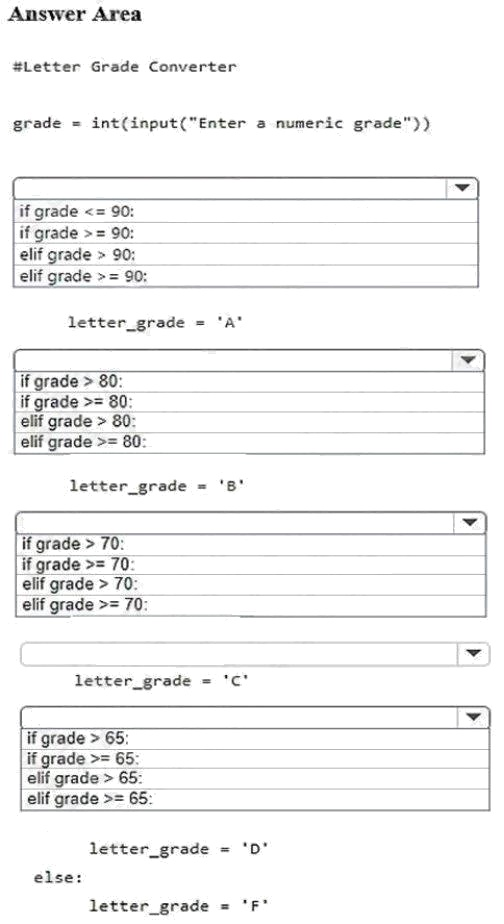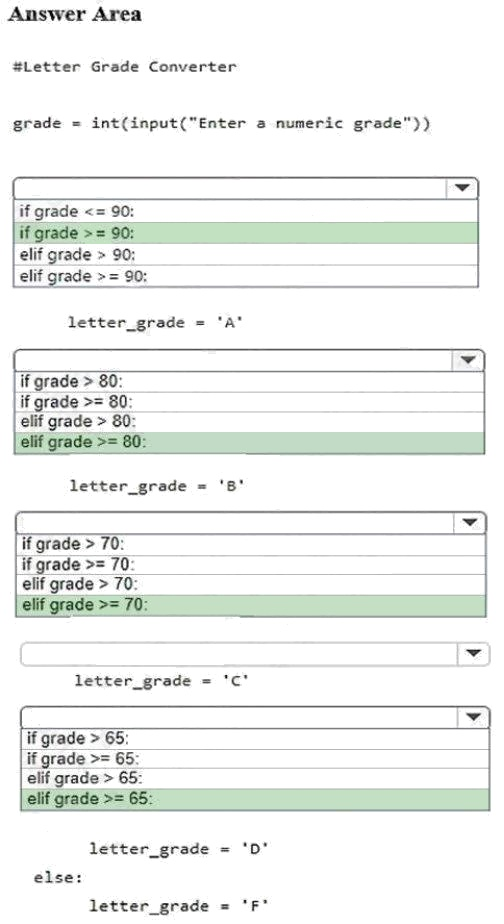Explanation:
References:
https://www.w3resource.com/python/python-if-else-statements.php

Discussions
0 / 1000

##### Question 15

You are writing code that generates a random integer with a minimum value of 5 and a maximum value of 11. Which two
functions should you use? Each correct answer presents a complete solution. (Choose two.)

• A. random.randint(5, 12)
• B. random.randint(5, 11)
• C. random.randrange(5, 12, 1)
• D. random.randrange(5, 11, 1)